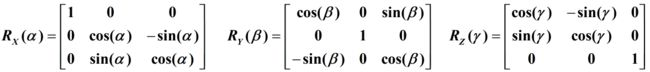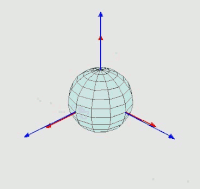• 输入矩阵是 3x3。 它仅适用于“ZYX”序列。 函数“dcm2angle”在 Airspace 工具箱中的 Matlab 2012b 中可用，它也启用其他序列。 但是，如果您没有访问该工具箱的权限，那么……这才是开始:-)。 包括一个例子。matlab
• - Algorithms for SINS * * Strap-down Inertial Navigation System 捷联惯导系统——介绍及算法(DCM) Introduction and Algorithms Outline 捷联惯导系统概述 姿态矩阵(DCM)微分方程的推导 方向余弦矩阵的求解:...

Lecture 10 -- Algorithms for SINS * * Strap-down Inertial Navigation System 捷联惯导系统——介绍及算法(DCM) Introduction and Algorithms Outline 捷联惯导系统概述 姿态矩阵(DCM)微分方程的推导 方向余弦矩阵的求解: 角增量算法 1.1*捷联惯导系统: 特点 方位轴 滚动轴 俯仰轴 陀螺和加速度计被直接“捆绑(strapped)”到载体上 没有物理的平台 对陀螺仪的角速度输出进行积分，获取载体的姿态信息. 加速度计的输出需要变换到导航坐标系中. 对导航坐标系中的加速度分量进行补偿并积分，获取载体的速度和位置信息. z, 方位轴 x, 滚动轴 y, 俯仰轴 1.2 姿态变换 加速度需要变换: C 包含姿态信息. 也可用欧拉角、四元数等表示姿态 1.3 SINS的示意框图 计算载体的姿态信息 姿态信息 计算机 沿载体轴的 加速度输出 沿载体轴的 角速率输出 惯性元件 对加速度分量进行坐标变换 沿地理坐标 系各轴的加速度 导航计算 显示 姿态 位置 速度 对地理坐标系进行 修正 数学平台 Outline 捷联惯导系统概述 姿态矩阵(DCM)微分方程的推导 方向余弦矩阵的求解: 角增量算法 z, 方位 x, 滚动 y, 俯仰 2.1 方向余弦矩阵 咋来的？ S1 设 S1 为导航坐标系，其单位坐标矢量为 i, j 和 k S2 S2 为载体坐标系, 其单位坐标矢量为 i’, j’ 和 k’ 2.1 方向余弦矩阵 C 为从 S2 到 S1 的方向余弦矩阵，即: S2 S1 其中 载体的旋转导致 C 发生变化 2.2 方向余弦矩阵的导数 so 设载体坐标系相对于导航坐标系的角速度为表示在载体坐标系中 则 类似的 则 2.3 方向余弦矩阵微分方程 -- 斜 对称矩阵 --- 其解取决于 C(0) 和载体的转动角速度的变化规律 Outline 捷联惯导系统概述 姿态矩阵(DCM)微分方程的推导 方向余弦矩阵的求解: 角增量算法 3.1 方向余弦矩阵微分方程 记初始时刻的地理坐标系为 G0 , 则有 其中 则 记 where (角增量) and denote (向量的模) 3.2*毕-卡解 ------ 毕-卡形式的解 可记为: 近似计算: 3.3*角增量算法 方程的解 各阶近似: 1st : 2nd : ……….. 许多类型的陀螺仪可用内部硬件完成对角速率的积分，从而直接输出角增量. 3.6 地理坐标系的修正 E N ζ 0 T E N ζ 3.7 算例 某捷联惯导系统在 n 时刻, 其载体坐标系和惯性坐标系重合. 然后从时刻 n 到时刻 n+1, 沿着载体三个轴的三个陀螺仪 X, Y, Z 的角增量输出分别为 0.002, 0.004 和 0.006 (rad); 请利用基于Peano-Paker 解的一阶角增量算法计算时刻 n+1 载体和惯性坐标系之间的方向余弦矩阵.

展开全文• 给定从 XYZ 到 xyz 的正交变换的方向余弦矩阵为 Q。求此变换的欧拉角 fi 、 theta 和 psi 。 有关更多示例，请访问www.smallsats.orgmatlab
• 主要讲述的是方向余弦矩阵，该文档能够快速让人理解DCM的原理，对apm里的DCM算法有更深理解
• 在工业机器人坐标变换中，旋转矩阵与欧拉角有几个概念比较容易混淆，根据自己的理解整理如下，如有问题，欢迎指正因为欧拉角相对旋转矩阵更为直观，直接用绕坐标轴的旋转可以得到，因此将旋转矩阵转换为欧拉角会方便...

在工业机器人坐标变换中，旋转矩阵与欧拉角有几个概念比较容易混淆，根据自己的理解整理如下，如有问题，欢迎指正

因为欧拉角相对旋转矩阵更为直观，直接用绕坐标轴的旋转可以得到，因此将旋转矩阵转换为欧拉角会方便分析

在位姿变换相关资料中(可参照 J. J. Craig 《Introduction to Robotics》)，位姿矩阵的描述如下上面这样的位姿描述，指的是主动旋转矩阵，即向量或子坐标系绕坐标轴主动旋转的旋转矩阵，也是机器人坐标变换通常会采取的旋转方式，写成 matlab 函数如下(a输入‘X’,'Y','Z'，B输入旋转角度)

function y = Rot3( a ,B )

switch a

case 'X'

y=[1,0,0;0,cos(b),-sin(b);0,sin(b),cos(b)];

case 'Y'

y=[cos(b),0,sin(b);0,1,0;-sin(b),0,cos(b)];

case 'Z'

y=[cos(b),-sin(b),0;sin(b),cos(b),0;0,0,1];

end

与此区分的是被动旋转矩阵，向量或子坐标系不变，基坐标系旋转的旋转矩阵，这类被动旋转矩阵与上面的三个矩阵互为转置(互为逆矩阵，对于旋转矩阵是等价的)，也可以理解为被动旋转矩阵让向量或子坐标系旋转到基坐标系位置，而 matlab 中的 dcm2angle，angle2dcm 均对应的是被动旋转：

Mat_t = [cos(pi/3),-sin(pi/3),0;sin(pi/3),cos(pi/3),0;0,0,1];

[ra,rb,rc]=dcm2angle(Mat_t,'ZYX');

%此处 ra 为 -60 度，可见 Matlab 中采用的是被动旋转，sin的符号和主动旋转矩阵相反

因此，在采用matlab 中 dcm2angle 计算欧拉角时，需要注意对旋转矩阵进行转置(R3')，以‘ZYX’欧拉角为例：

R3 = Rot3('Z',20)*Rot3('Y',60)*Rot3('X',40)

[ra,rb,rc]=dcm2angle(R3','ZYX');

M3 = Rot3r('Z',ra)*Rot3r('Y',rb)*Rot3r('X',rc)

d=[ra,rb,rc]./pi.*180

这样，计算结果  d = 20.0000   60.0000   40.0000  ，与常用主动旋转矩阵一致，R3与M3也一致，如果R3‘转置去掉，改为

[ra,rb,rc]=dcm2angle(R3,'ZYX');

则结果为从R3转换到基坐标的欧拉角，对应M3为R3的逆矩阵

需要注意的是，主动/被动旋转不等同于基坐标系、连体坐标系的变换，在主动旋转矩阵下，仍然要区分按照固定基坐标系坐标轴，还是按照旋转后的连体坐标新坐标轴旋转，按照右乘连体左乘基的方式进行计算

展开全文• 最近用matlab和Eigen库中的一些旋转矩阵（方向余弦矩阵）、四元数和欧拉角之间的转换和绘图，弄得我有些头疼，把遇到的问题记录一下，以防以后又脑阔疼....有不同的理解可以再评论区批评指正～ 主要问题有两个 1、...

最近用matlab和Eigen库中的一些旋转矩阵（方向余弦矩阵）、四元数和欧拉角之间的转换和绘图，弄得我有些头疼，把遇到的问题记录一下，以防以后又脑阔疼....有不同的理解可以再评论区批评指正～

主要问题有两个

1、matlab工具箱自带的的姿态转换函数定义有歧义（quat2eul（）和quat2angle( )定义不一样），会导致转换出来的欧拉角结果出错；

2、eigen库中使用欧拉角转换eulerAngles（）时，得到的结果每轴相差180度(看起来不太对，其实真正结果时对的)

• 定义

首先要明确一下欧拉角、旋转矩阵、四元数的在表示旋转时的定义，参考：

https://zh.wikipedia.org/wiki/%E6%AC%A7%E6%8B%89%E8%A7%92

https://zh.wikipedia.org/wiki/%E6%97%8B%E8%BD%AC%E7%9F%A9%E9%98%B5

https://zh.wikipedia.org/wiki/%E5%9B%9B%E5%85%83%E6%95%B8

很可惜地，對於夾角的順序和標記，夾角的兩個軸的指定，並沒有任何常規。科學家對此從未達成共識。每當用到歐拉角時，我們必須明確的表示出夾角的順序，指定其參考軸。

这就是欧拉角很烦人的原因...很多情况下，我感觉在说欧拉角的时候，并没有提到其的旋转轴的顺序，故在画图的时候一般使用欧拉角来表示比较直观，就非常非常的头疼（也可能是我太菜了orz）...

所以，对于姿态表示欧拉角是不唯一的，而旋转矩阵和四元数是唯一的！

欧拉角的表示方式有24种，即2×（3*2*2）种，2代表内旋和外旋，即是固定坐标轴转还是按转动后的坐标轴转的；3代表第一次旋转的3个轴向，接着2代表除了上次的那个轴外的另外两个轴向，还有一个2也是代表除了上次的那个轴外的另外两个轴向。

内旋(intrinsic rotations) = 旋转轴(rotated axis)

外旋(extrinsic rotations) = 固定轴(static/fixed axis)

https://zhuanlan.zhihu.com/p/85108850

所以我们在使用欧拉角转旋转矩阵和四元数的时候，要首先分清时内旋还是外旋，然后在分清其旋转轴向...比如该图代表的就是按转动后的坐标轴（蓝色的）计算欧拉角的，即为内旋

在一般的导航定位，比如无人车、无人机等的欧拉角表示，如果没有说明，则很可能是内旋的ZYX顺序。

• Matlab函数转换

一般情况下，我们做完位姿解算，会使用matlab画出误差曲线来判断结果是否准确，这个时候一般用欧拉角来绘图比较直观，然而在绘图的时候，有时候会感觉画出来的欧拉角很奇怪，比如欧拉角上下相反...

在MATLAB工具包使用了两种不同的方式来表示四元数，在空航天工具箱中使用的四元数惯例本质上是其机器人学工具箱中使用的四元数惯例的共轭。 有关这些区别的更多详细信息，请参见以下链接：

Robotics Toolbox的是quat2eul( )

Aerospace Toolbox的是quat2angle( )

并且在matlab中，四元数的定义是a+bi+cj+dk，即w、x、y、z.

https://ww2.mathworks.cn/help/robotics/ref/quaternion.html

一般我们定义的四元数是按右手法则的，即拇指朝向旋转轴（xyz的方向），四指指向旋转方向（w代表旋转大小），这和Robotics Toolbox的是quat2eul( ) 的函数是吻合的，而Aerospace Toolbox的是quat2angle( )则是左手法则，故差一个共轭！

这些在使用相应函数时都要注意。

另外，这个工具箱有时候不好安装，要激活matlab的权限，对有的破解版来说比较麻烦，所以我觉得还是直接自己写一个转换代码比较方便T_T

这里代码的定义是一般无人机使用的欧拉角的定义，即内旋的ZYX

function [rpy] = q2euler(q)
% [qx qy qz qw] --> [roll, pitch, yaw]

qx = q(1);
qy = q(2);
qz = q(3);
qw = q(4);

roll = atan2(2*(qw*qx+qy*qz), 1-2*(qx*qx+qy*qy));
pitch = asin(2*(qw*qy-qz*qx));
yaw = atan2(2*(qw*qz+qx*qy), 1-2*(qy*qy+qz*qz));

rpy = [roll, pitch, yaw];
end
function [rpy] = c2euler(c)
%UNTITLED2 此处显示有关此函数的摘要
%   此处显示详细说明
rpy(1) = atan2(c(3,2),c(3,3));
rpy(2) = atan2(-c(3,1),sqrt(c(3,2)^2+c(3,3)^2));
rpy(3) = atan2(c(2,1),c(1,1));
end

具体请参考

https://www.cnblogs.com/tiandsp/p/10733607.html

• Eigen库转换

在Eigen库中有互相转换的函数，见如下代码示例

旋转矩阵转欧拉角时，若该旋转矩阵是之前由欧拉角转换来的，想得到之前的欧拉角的值，则旋转轴的顺序必须一致，如从ZYX顺序来的，则使用eulerAngles（）是得用ZYX顺序，即（2,1,0），得到的为ypr的值。

注意，Eigen库中eulerAngles（）是默认内旋的方式

* \returns the Euler-angles of the rotation matrix \c *this using the convention defined by the triplet (\a a0,\a a1,\a a2)
*
* Each of the three parameters \a a0,\a a1,\a a2 represents the respective rotation axis as an integer in {0,1,2}.
* For instance, in:
* \code Vector3f ea = mat.eulerAngles(2, 0, 2); \endcode
* "2" represents the z axis and "0" the x axis, etc. The returned angles are such that
* we have the following equality:
* \code
* mat == AngleAxisf(ea, Vector3f::UnitZ())
*      * AngleAxisf(ea, Vector3f::UnitX())
*      * AngleAxisf(ea, Vector3f::UnitZ()); \endcode
* This corresponds to the right-multiply conventions (with right hand side frames).
*
* The returned angles are in the ranges [0:pi]x[-pi:pi]x[-pi:pi].

int main(int argc, char **argv)
{
Eigen::Vector3d rpy_raw, ypr;
rpy_raw << 30, 60, 150;
rpy_raw = rpy_raw * M_PI / 180;

Eigen::Matrix3d c;

//输入部分为YPR,所以转换的输出也是YPR
c = Eigen::AngleAxisd(rpy_raw, Eigen::Vector3d::UnitZ()) * Eigen::AngleAxisd(rpy_raw, Eigen::Vector3d::UnitY()) * Eigen::AngleAxisd(rpy_raw, Eigen::Vector3d::UnitX());
ypr = c.eulerAngles(2, 1, 0);

cout << ypr.transpose() * 180 / M_PI << endl;

return 0;
}

这个是按ZYX转的

下面这个是按照XYZ方式旋转的

//主函数
int main(int argc, char **argv)
{

Eigen::Vector3d rpy_raw, rpy_1;
rpy_raw << 30, 60, 150;
rpy_raw = rpy_raw * M_PI / 180;

Eigen::Matrix3d c;

//输入部分为RPY,所以转换的输出也是RPY
c = Eigen::AngleAxisd(rpy_raw, Eigen::Vector3d::UnitX()) * Eigen::AngleAxisd(rpy_raw, Eigen::Vector3d::UnitY()) * Eigen::AngleAxisd(rpy_raw, Eigen::Vector3d::UnitZ());
// c = Eigen::AngleAxisd(rpy_raw, Eigen::Vector3d::UnitZ()) * Eigen::AngleAxisd(rpy_raw, Eigen::Vector3d::UnitY()) * Eigen::AngleAxisd(rpy_raw, Eigen::Vector3d::UnitX());
rpy_1 = c.eulerAngles(0, 1, 2);

cout << rpy_1.transpose() * 180 / M_PI << endl;

return 0;
}

若时四元数的话，只需要先把四元数转成旋转矩阵，再转成欧拉角即可

Eigen::Quaterniond q;
Eigen::Matrix3d c;
c = q.toRotationMatrix();

回到最初的问题，为什么使用eulerAngles（）有时候得到的欧拉角会相差180度

代码如下

int main(int argc, char **argv)
{
Eigen::Vector3d rpy_raw;
rpy_raw << -1, 2, 3;
rpy_raw = rpy_raw * M_PI / 180;

Eigen::Matrix3d c;
c = Eigen::AngleAxisd(rpy_raw, Eigen::Vector3d::UnitZ()) * Eigen::AngleAxisd(rpy_raw, Eigen::Vector3d::UnitY()) * Eigen::AngleAxisd(rpy_raw, Eigen::Vector3d::UnitX());
Eigen::Vector3d rpy_tran = c.transpose().eulerAngles(2, 1, 0) / M_PI * 180;
std::cout << rpy_tran << " " << rpy_tran << " " << rpy_tran << std::endl;

return 0;

输出结果

-178.896 -178.055 176.964

正确的结果应该时-1,2,3的，我们转换得到的结果貌似每轴和正确结果都相差180度

回到eulerAngles（）定义中去，有一句话

The returned angles are in the ranges [0:pi]x[-pi:pi]x[-pi:pi].

即row的角度范围时【0-180°】，当我们row小于0时，Eigen库会把YZ两轴旋转180度，从而达到让X轴旋转角度大于0°的目的，各位可以试一试按ZYX的方式旋转，将ZY分别旋转180度，原本X为负的旋转角就变成了正的！

这就是Eigen库输出的欧拉角看起来不太对的原因了！其实我们用到欧拉角，主要还是在画图中，比较直观，计算姿态的话直接用旋转矩阵和四元数，就没有这么多麻烦事了～

展开全文Eigen 欧拉角 旋转矩阵 matlab
• 欧拉角，四元数和方向余弦矩阵三者之间相互转换的代码，采用北-东-地坐标系欧拉角
• 学习过程中涉及欧拉角和旋转矩阵的转换，索性整理学习一下欧拉角四元数和旋转矩阵的概念以及matlab中的互相转换本文摘自各大课本，博客，自己学习...1旋转矩阵(方向余弦矩阵)当确定一个点在空间中的位置后，要确定...

学习过程中涉及欧拉角和旋转矩阵的转换，索性整理学习一下欧拉角四元数和旋转矩阵的概念以及matlab中的互相转换

本文摘自各大课本，博客，自己学习整理使用，侵删

MATLAB矩阵乘法从左到右依次相乘

用R表示旋转矩阵。

yaw(偏航) pitch(俯仰) roll(横滚)分别表示Z　Ｙ　Ｘ轴的转角。

q=[q0,q1,q2,q3]’表示单位四元数。

1旋转矩阵(方向余弦矩阵)

当确定一个点在空间中的位置后，要确定其姿态才能完全定义该点的位姿。所以采用坐标系{B}相对于坐标系{A}的描述来表示物体姿态，通俗的讲，是坐标系{B}的各个轴分别与参考坐标系{A}的各个轴的余弦值构成的3×3矩阵，称为旋转矩阵。欧拉角

1、内旋和外旋内在旋转与外在旋转的转换关系：互换第一次和第三次旋转的位置则两者结果相同。例如Z-Y-X旋转的内部旋转和X-Y-Z旋转的外部旋转的旋转矩阵相同。

一、绕定轴X-Y-Z旋转(RPY角)(外旋)

假设两个坐标系A和B，二者初始时完全重合。

过程如下：B绕A的X轴旋转γ角，再绕A的Y轴旋转β角，最后绕A的Z轴旋转α角，完成旋转。整个过程，A不动B动。旋转矩阵的计算方法如下：R = Rz * Ry *Rx，乘法顺序：从右向左，依次旋转X轴Y轴Z轴其中，cα = cosα，sα = sinα，矩阵相乘，结果如下：二、绕动轴Z-Y-X旋转(Euler角)(内旋)

过程如下：B绕B的Z轴旋转α角，再绕B的Y轴旋转β角，最后绕B的X轴旋转γ角，完成旋转。整个过程，A不动B动。

旋转矩阵的计算方法如下：R = Rz * Ry *Rx。乘法顺序：从左向右欧拉角的表示方式比较直观，但是有几个缺点：

(1) 欧拉角的表示方式不唯一。给定某个起始朝向和目标朝向，即使给定yaw、pitch、roll的顺序，也可以通过不同的yaw/pitch/roll的角度组合来表示所需的旋转。比如，同样的yaw-pitch-roll顺序，(0,90,0)和(90,90,90)会将刚体转到相同的位置。这其实主要是由于万向锁(Gimbal Lock)引起的

(2) 欧拉角的插值比较难。

(3) 计算旋转变换时，一般需要转换成旋转矩阵，这时候需要计算很多sin, cos，计算量较大。

欧拉角转旋转矩阵

在计算坐标变换时，旋转更方便的表示形式是旋转矩阵(Rotation Matrix)。三维空间的旋转矩阵可以表示成3×3的矩阵，将欧拉角转换为旋转矩阵的计算方式如下，假设欧拉角yaw、pitch、roll的角度为alpha, beta, gamma，则旋转矩阵可以计算如下：eul2rotm(eul)中，默认旋转顺序是ZYX,而矩阵相乘顺序是从右到左，先X再Y后Z，

即：若eul(Z Y X)=eul(angle1 angle2 angle3)，则eul2rotm(eul,sequence)=Rot(Z,angle1)*Rot(Y,angle2)*Rot(X,angle3).rotm2eul()

计算单位为弧度制，计算结果的顺序对应欧拉角旋转轴顺序

% Z Y X

eul=[0 pi/2 0];

yaw=0;

pitch=pi/2'

roll=0;

R_x=[1 0 0;

0 cos(roll) -sin(roll);

0 sin(roll) cos(roll)];

R_y=[cos(pitch) 0 sin(pitch);

0 1 0;

-sin(pitch) 0 cos(pitch)];

R_z=[cos(yaw) -sin(yaw) 0;

sin(yaw) cos(yaw) 0;

0 0 1];

R=R_z*R_y*R_x;%欧拉角转旋转矩阵

R2=angle2dcm(eul,'ZYX');%由欧拉角转方向余弦矩阵

R2=eul2rotm(eul,'ZYX');%欧拉角转旋转矩阵

四元数(后续补充)

一篇介绍四元数的文章

在Matlab里，可以用quatmultiply计算四元数乘法，用quatinv来计算四元数的逆，用quatconj来计算四元数的共轭。四元数的旋转和旋转矩阵的旋转可以由以下matlab代码验证：

% Matlab code by MulinB, Aerospace Toolbox is needed

pt = [10,20,30]; % point coordinate

yaw = 45;

pitch = 30;

roll = 60;

q = angle2quat(yaw/180*pi,pitch/180*pi,roll/180*pi);

R = angle2dcm(yaw/180*pi,pitch/180*pi,roll/180*pi);

pt1 = R*pt';

pt2 = quatmultiply(quatconj(q), quatmultiply([0,pt],q)); % NOTE the order

disp(pt1');disp(pt2(2:4));

从上述代码里也可以看到四元数和欧拉角和dcm的转换，在matlab里可以很方便的用quat, dcm, angle之间的转换来任意互转。另外，从四元数计算axis和angle，可以用以下代码计算：

% Matlab code by MulinB, Compute the axis and angle from a quaternion

function [axis, theta] = quat2axisangle(q)

theta = acos(q(1)) * 2;

axis = q(2:4)/sin(theta/2);

总结：

转欧拉角：

旋转矩阵转欧拉角

[r2,r2,r3]=dcm2angle(R, S)

eul=rotm2rul(R,S)

注：得到的结果为弧度，若需要角度需进一步转化

四元数转欧拉角

[r1,r2,r3]=quat2angle([q0 q1 q2 q3]，S)

注：S 的选择有12种，【‘ZYX’,‘ZYZ’,‘ZXY’,‘ZXZ’,‘YXZ’,‘YXY’,‘YZX’,‘YZY’,‘XYZ’,‘XYX’,‘XZY’,‘XZX’】

S 默认 ‘ZYX’

转旋转矩阵

四元数转旋转矩阵

R=quat2dcm([q0 q1 q2 q3])

欧拉角转旋转矩阵

R=angle2dcm(r1,r2,r3,S);

R=angle2dcm(yaw/180pi,pitch/180pi,roll/180*pi)

注：根据欧拉角是弧度/角度，选择以上操作

转四元数

旋转矩阵转四元数

q =dcm2quat®；

欧拉角转四元数

q=angle2quat(r1,r2,r3，S)；

clear all;

close all;

clc;

%欧拉角

x = 0.5;

y = 0.6;

z = 0.7;

Ang1 = [x y z];

%欧拉角转旋转矩阵

Rx = [1 0 0;

cos(x) -sin(x);

sin(x) cos(x)];

Ry = [cos(y) 0 sin(y);

1 0;

-sin(y) 0 cos(y)];

Rz = [cos(z) -sin(z) 0;

sin(z) cos(z) 0;

0 1];

R = Rz*Ry*Rx;

R1 = R;

%旋转矩阵转欧拉角

x = atan2(R(3,2),R(3,3));

y = atan2(-R(3,1),sqrt(R(3,2)^2+R(3,3)^2));

z = atan2(R(2,1),R(1,1));

Ang2 = [x y z];

%旋转矩阵转四元数

t=sqrt(1+R(1,1)+R(2,2)+R(3,3))/2;

q=[t (R(3,2)-R(2,3))/(4*t) (R(1,3)-R(3,1))/(4*t) (R(2,1)-R(1,2))/(4*t)];

Q1 = q;

%四元数转旋转矩阵

R=[ 2*q(1).^2-1+2*q(2)^2 2*(q(2)*q(3)-q(1)*q(4)) 2*(q(2)*q(4)+q(1)*q(3));

2*(q(2)*q(3)+q(1)*q(4)) 2*q(1)^2-1+2*q(3)^2 2*(q(3)*q(4)-q(1)*q(2));

2*(q(2)*q(4)-q(1)*q(3)) 2*(q(3)*q(4)+q(1)*q(2)) 2*q(1)^2-1+2*q(4)^2];

R2 = R;

%欧拉角转四元数

q = [cos(x/2)*cos(y/2)*cos(z/2) + sin(x/2)*sin(y/2)*sin(z/2) ...

sin(x/2)*cos(y/2)*cos(z/2) - cos(x/2)*sin(y/2)*sin(z/2) ...

cos(x/2)*sin(y/2)*cos(z/2) + sin(x/2)*cos(y/2)*sin(z/2) ...

cos(x/2)*cos(y/2)*sin(z/2) - sin(x/2)*sin(y/2)*cos(z/2)];

Q2 = q;

%四元数转欧拉角

x = atan2(2*(q(1)*q(2)+q(3)*q(4)),1 - 2*(q(2)^2+q(3)^2));

y = asin(2*(q(1)*q(3) - q(2)*q(4)));

z = atan2(2*(q(1)*q(4)+q(2)*q(3)),1 - 2*(q(3)^2+q(4)^2));

Ang3 = [x y z];

Ang1

Ang2

Ang3

R1

R2

Q1

Q2

展开全文• 一、欧拉角转四元数（常用来初始化四元数） 按Z-Y-X的旋转变换顺序有： 二、四元数与旋转矩阵（常用来作坐标变换） 1.b系到R系的坐标变换...三、欧拉角转方向余弦矩阵 由以上两式可得，经三次基本旋转对应的坐标变换
•matlab
• 欧拉角转换成方向余弦矩阵欧拉角有12种旋转顺序分别为 - 1 X-Z-Y - 2 Y-X-Z - 3 Y-Z-X - 4 Z-X-Y - 5 Z-Y-X - 6 X-Y-X - 7 X-Z-X …. 每种旋转顺序可以分解为3次旋转，每次旋转或者为绕X轴，或者绕...欧拉角 方向余弦矩阵 转换
• 衡量两个向量x、y的相关性(夹角的余弦) MATLAB 代码 ，余弦相似度也被⼀些迁移学习研究所使用。
• 余弦相似度计算matlab代码计算余弦相似度 张伯超 该脚本将从免疫数据库中读取数据并计算不同特征样本之间的余弦相似度。 用法 -d name of database -s name of subject -f field of the columns used to separate ...
• 用法： mO=smoothc(mI,Nr,Nc) mI=输入矩阵Nr=平滑函数中包含的行数Nc=平滑函数中包含的列数mO=输出数组，平滑 旨在作为使用内核平滑图像的示例。 只需少量更改，用户就可以实现另一个内核。matlab
• 上两篇文章我们讲了旋转矩阵和欧拉角，可知欧拉角是可以由旋转矩阵转化而来。 那么怎么从欧拉角转化为旋转矩阵呢？欧拉角（Euler angles）与旋转矩阵（Rotation Matrix）假设坐标系1的欧拉角yaw（Azimuth）、pitch...
• Matlab中常见数学函数的使用_数学_自然科学_专业资料。. 给自己看的---Matlab ...(x) 反三角函数 atan(x) acot(x) asec(x) acsc(x) sinh(x) cosh(x)......(x), x,n) 20 在 MATLAB 中没有直接求隐函数导数的命令,但是...
• 通过与坐标系的任何方向相关的那个点的描述性应力矩阵，可以传达关于该点应力状态的大量信息。 该程序通过代数运算得出其中一些参数。matlab
• 上一篇博文提到了离散傅里叶变换，地址如下：http://blog.sina.com.cn/s/blog_7445c2940102wcdj.html其实，离散余弦变换(DCT)就是离散傅里叶变换(DFT)的一部分，那么既然已经有了DFT，为什么还需要DCT呢？...
• 1. 几种相似度1.1 Jaccard系数杰卡德...1.2 余弦相似度余弦相似度，又称为余弦相似性，是通过计算两个向量的夹角余弦值来评估他们的相似度。对于二维空间，根据向量点积公式，显然可以得知：设向量 A = (A1,A2,.....
• close all;clear all;clc; A=[1 1 1 1;2 2 2 2;3 3 3 3] s=size(A); M=s(1); N=s(2);...P=dctmtx(M) %离散余弦变换矩阵 Q=dctmtx(N) %离散余弦变换矩阵 B=P*A*Q' %离散余弦变换 输出结果如下： ...dctmtx函数
• 若微信排版有问题,请点击阅读原文查看MATLAB01:基本的数学运算与矩阵运算MATLAB基本语法变量变量名保留变量不适合做变量名变量不应当覆盖内置函数变量类型数字型变量的显示格式MATLAB命令行使用MATLAB进行数字运算...
• 将任何阶 (>0) 的矩阵 3D 张量（欧几里得或笛卡尔... % % Tranmatrix - 变换矩阵，包含方向的 3x3 矩阵% 旧坐标系和新坐标系之间的余弦。 % % 参数：（输出） % otr - 转换后的输出张量； 具有与输入张量相同的维度。matlab
• Matlab操作矩阵的相关方法

千次阅读 2021-04-23 19:06:11
Matlab操作矩阵的相关方法下面这篇文章主要是对吴恩达老师机器学习中matlab操作的一个整理和归纳一、基本操作1.生成矩阵(ones、zeros)A = [1 2;3 4;5 6] #生成3行4列的矩阵B = [1 2 3] #B就是一个行向量C = [1;2;3] ...
• 问题描述：matlab 一个向量和矩阵中各行向量的夹角余弦值比如A=(1,2,3,4,5)B={1,2,3,4,5;6,7,8,9,10;11,12,13,14,15;16,17,18,19,20}分别求向量A与矩阵B中各行向量的夹角余弦值并输出最大的夹角余弦值1个回答分类：...
• 大概从去年冬天就开始接触姿态解算的内容了，中间走过很多弯路，当时一度迷糊在理论中，那时前辈让先学习方向余弦矩阵，但实际上，这个实际不常用。不过关于旋转矩阵的那个不正交矫正的原理倒是可以看看，非常巧妙。...经验分享 程序人生
• MATLAB在图像相似度分析中的应用杨涛;董洁;白倩靖【期刊名称】《电脑迷》【年(卷),期】2017(000)008【摘要】近日,微博热门话题"被烤鱼征服找到亲生父母"引起广泛关注,最终主人公通过图像识别技术成功寻找到自己的...
• 向量与矩阵矩阵矩阵余弦相似度 很简单，将公式套上就行了。 python 代码 import numpy as np a = np.array([[1, 2, 1, 2, 3, 5, 6, 2]]) b = np.array([ [1, 2, 1, 2, 3, 5, 6, 2], [1, 2, 1, 2, 3, 5,...余弦相似度 向量相似度 矩阵相似度
• matlab余弦相似度代码用于盲语音分离的定向稀疏滤波。 以下论文的 MATLAB 代码： K. Watcharasupat, AHT Nguyen, C. -H. Ooi 和 AWH Khong，“使用加权 Lehmer 均值进行不平衡语音混合盲分离的定向稀疏滤波”，......

matlab方向余弦矩阵matlab 订阅